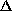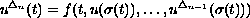Electron. J. Diff. Eqns., Vol. 2000(2000), No. 54, pp. 1-10.

### Smoothness of solutions of conjugate boundary-value problems on a measure chain Eric R. Kaufmann

Abstract:
In this paper we consider the n-th order-differential equation (often refered to as a differential equation on a measure chain)satisfying n-point conjugate boundary conditions. We show that solutions depend continuously and smoothly on the boundary values.

Submitted June 27, 2000. Published July 14, 2000.
Math Subject Classifications: 34B15, 34B99, 39A10, 34A99.
Key Words: Measure chain, initial-value problem, boundary-value problem

Show me the PDF file (138K), TEX file, and other files for this article.Eric R. Kaufmann Department of Mathematics and Statistics University of Arkansas at Little Rock Little Rock, Arkansas 72204-1099 USA e-mail: erkaufmann@athena.ualr.edu